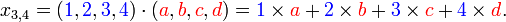# Activity 1-4

February 12, 2013

1. Download and save `ACT1-4_starter.xlsx`. Open it in Excel and go to the `OffsetExample2` sheet.
2. This sheet breaks `OffsetExample` from last class into multiple parts by first specifying the cells that we want to average. Change the values in each of the pink cells and observe what happens.
3. Fill in the orange cells to get identical results to the green and blue cells. All of the correct steps and functions are listed next to the cells. This is to give you practice with using array formulas, or formulas that are applied over a range of cells. Note two things:
• To make a formula fill a range of cells, you must press the Ctrl, Shift, and Enter keys at the same time. The formula will then have curly braces around it (like `{=OFFSET(B16:F16,I3-1,0)}`).
• To turn a row into a column, use the `TRANSPOSE` function, which must be entered as an array formula over a range of cells.

1. Go to the `MatrixMultiplication` sheet. You will find three lists: Grocery Store Prices, Bob's Grocery List, and Carol's Grocery List.
3. We can do the same thing using the `MMULT` function. Matrix Multiplication takes the rows of the first matrix, multiplies each element by the columns of the second matrix, and returns the sum for each row/column pair. The size of the matrices is important: the number of columns in the first matrix must be equal to the number of rows in the second matrix. Check out the example below (Figures from Wikipedia).Use the `MMULT` function to find Bob's food bill.
4. Now compute Carol's food bill, first by multiplying and then using the `MMULT` function. You will need to (1) use the `TRANSPOSE` function and (2) treat it as a array formula (Hint: use Ctrl + Shift + Enter; see the previous task).
5. Finally, we can compute Bob's and Carol's food bills at the same time with a single `MMULT` function. First, put both Bob's and Carol's grocery lists in a single matrix (the orange cells).
6. Use a single `MMULT` function for the range of blue cells to compute both bills at the same time.